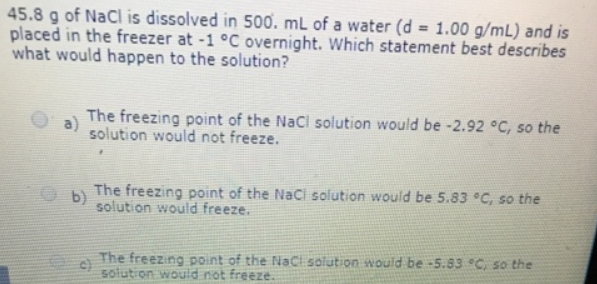# Problem: 45.8 g of NaCl is dissolved in 500, mL of a water (d = 1.00 g/mL) and is placed in the freezer at -1°C overnight. Which statement best describes what would happen to the solution? a) The freezing point of the NaCl solution would be -2.92°C, so the solution would not freeze. b) The freezing point of the NaCl solution would be 5.83°C, so the solution would freeze. c) The freezing point to the NaCl solution would be -5.83°C, so the solution on would not freeze.

🤓 Based on our data, we think this question is relevant for Professor Gary's class at HAWAII.

###### FREE Expert Solution###### Problem Details

45.8 g of NaCl is dissolved in 500, mL of a water (d = 1.00 g/mL) and is placed in the freezer at -1°C overnight. Which statement best describes what would happen to the solution?

a) The freezing point of the NaCl solution would be -2.92°C, so the solution would not freeze.

b) The freezing point of the NaCl solution would be 5.83°C, so the solution would freeze.

c) The freezing point to the NaCl solution would be -5.83°C, so the solution on would not freeze.